Logarithmic Differentiation - Type 2

Chapter 5 Class 12 Continuity and Differentiability
Concept wise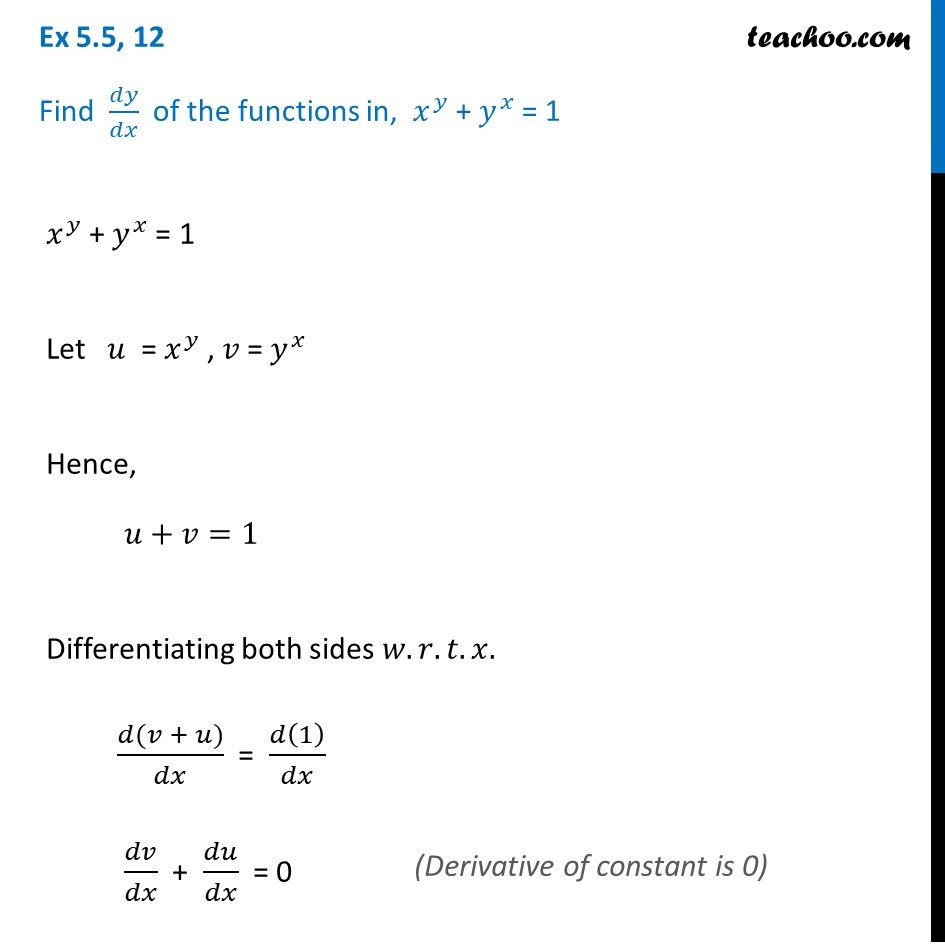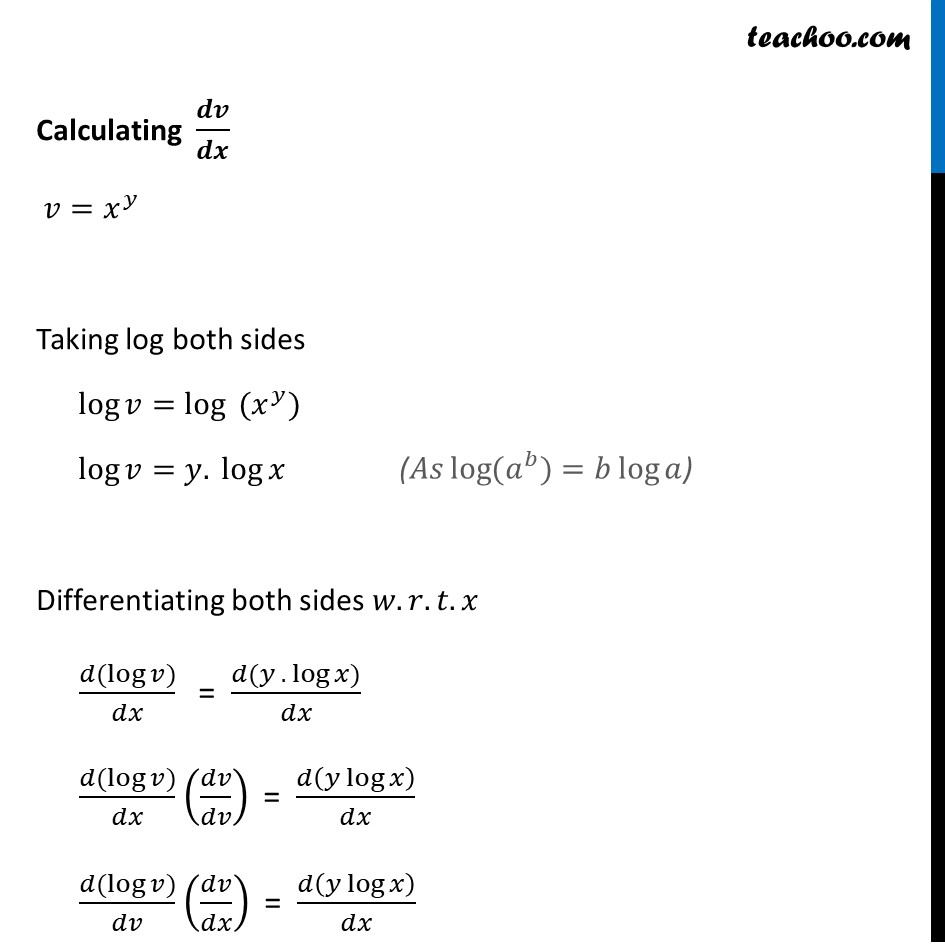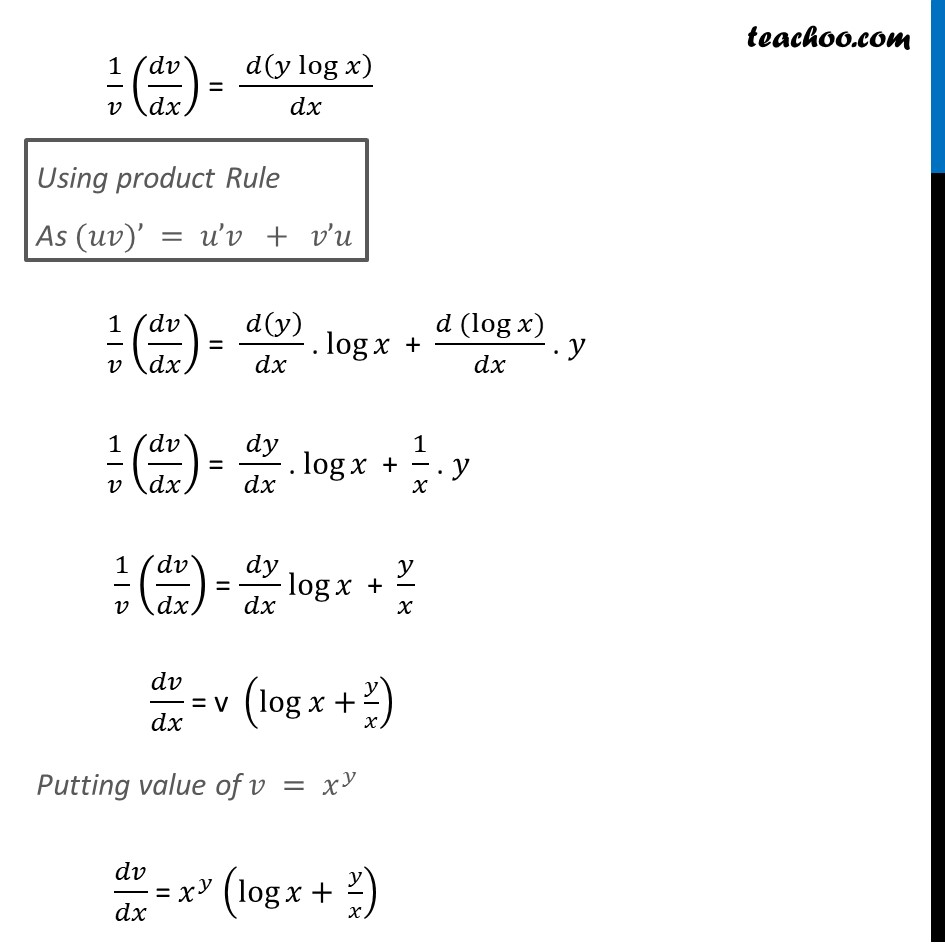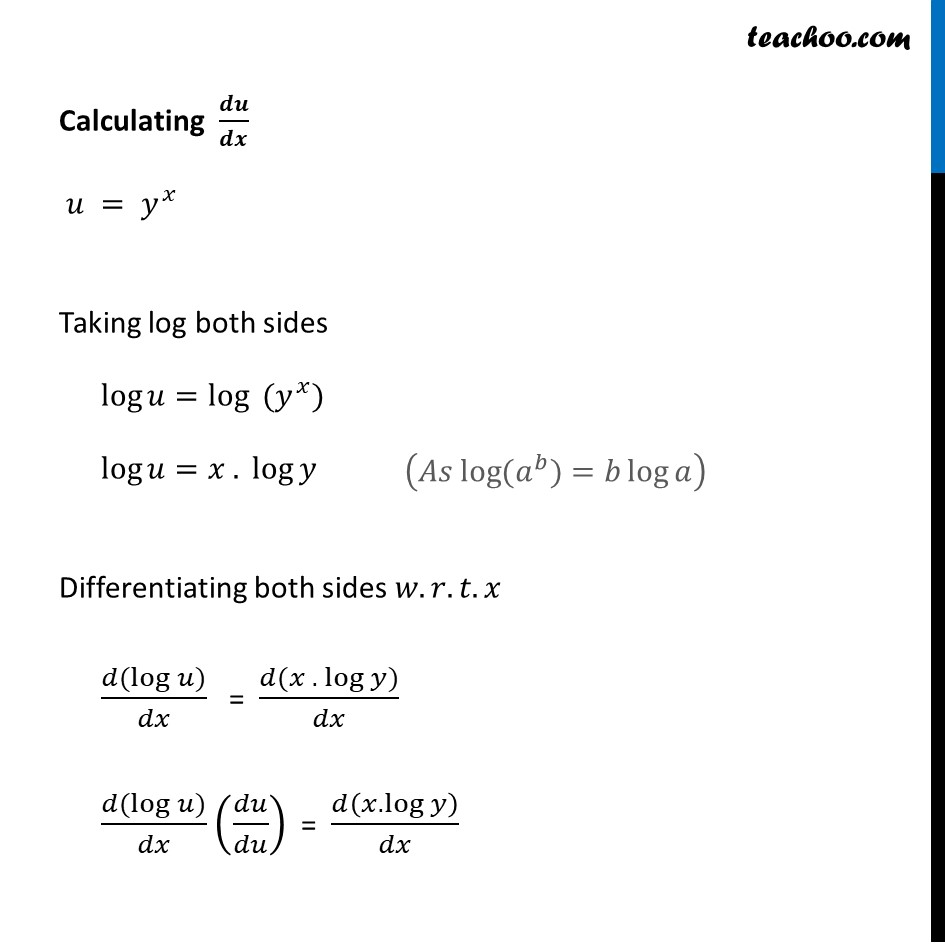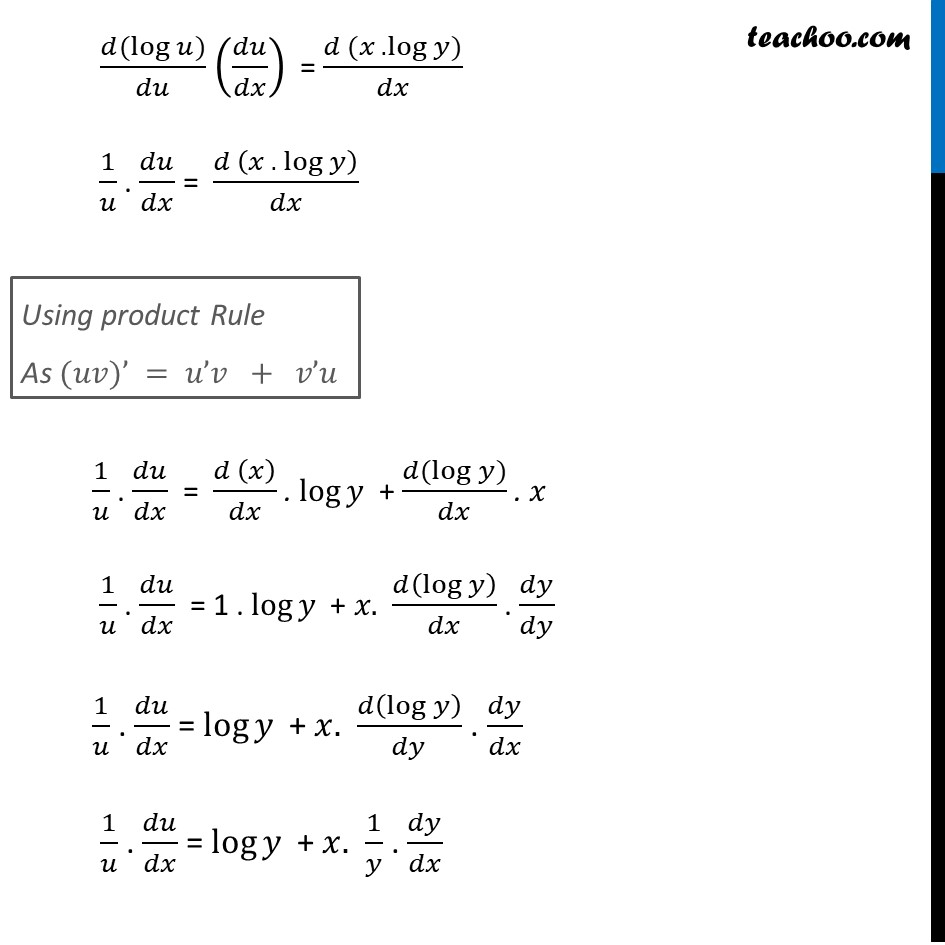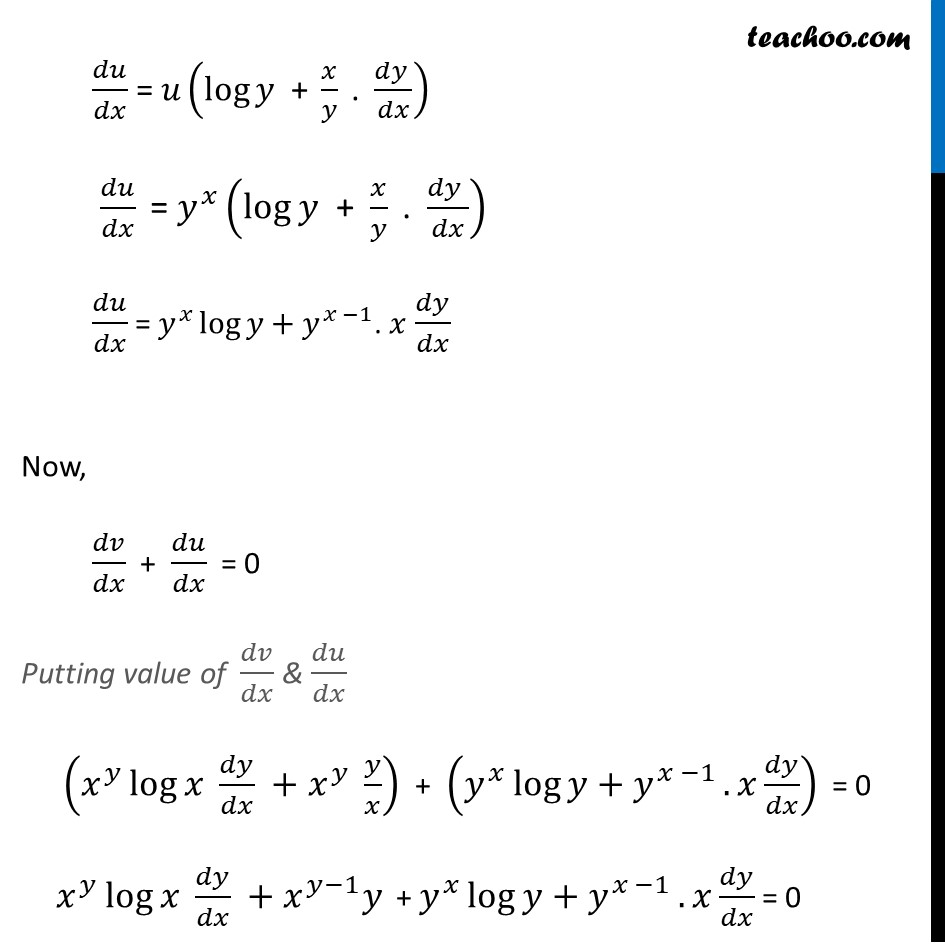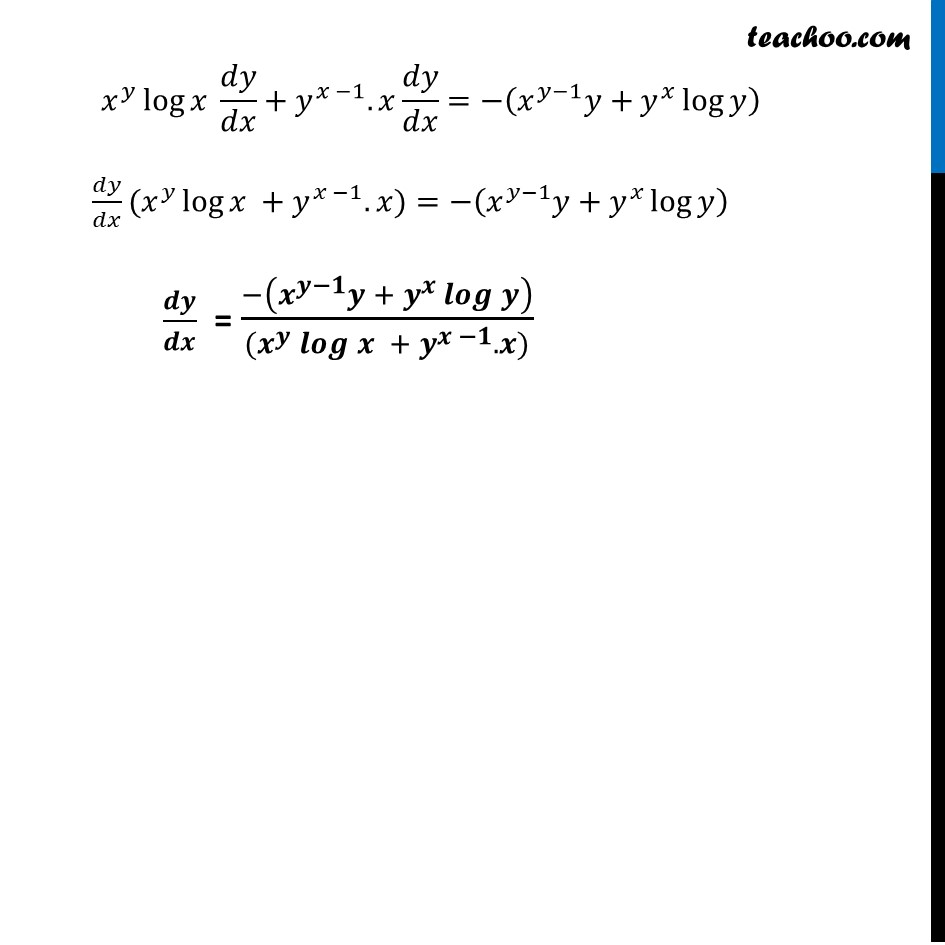Introducing your new favourite teacher - Teachoo Black, at only ₹83 per month

### Transcript

Ex 5.5, 12 Find 𝑑𝑦/𝑑𝑥 of the functions in, 𝑥^𝑦 + 𝑦^𝑥 = 1 𝑥^𝑦 + 𝑦^𝑥 = 1 Let 𝑢 = 𝑥^𝑦 , 𝑣 = 𝑦^𝑥 Hence, 𝑢+𝑣=1 Differentiating both sides 𝑤.𝑟.𝑡.𝑥. (𝑑(𝑣⁡〖+ 𝑢〗))/𝑑𝑥 = 𝑑(1)/𝑑𝑥 𝑑𝑣/𝑑𝑥 + 𝑑𝑢/𝑑𝑥 = 0 (Derivative of constant is 0) Calculating 𝒅𝒗/𝒅𝒙 𝑣=𝑥^𝑦 Taking log both sides log⁡𝑣=log⁡〖 (𝑥^𝑦)" " 〗 log⁡𝑣=〖𝑦. log〗⁡𝑥" " Differentiating both sides 𝑤.𝑟.𝑡.𝑥 (𝑑(log⁡𝑣))/𝑑𝑥 = (𝑑(𝑦 . log⁡𝑥))/𝑑𝑥 (𝑑(log⁡𝑣))/𝑑𝑥 (𝑑𝑣/𝑑𝑣) = 𝑑(𝑦 log⁡𝑥 )/𝑑𝑥 (𝑑(log⁡𝑣))/𝑑𝑣 (𝑑𝑣/𝑑𝑥) = 𝑑(〖𝑦 log〗⁡𝑥 )/𝑑𝑥 1/𝑣 (𝑑𝑣/𝑑𝑥) = ( 𝑑(〖𝑦 log〗⁡𝑥 ))/𝑑𝑥 1/𝑣 (𝑑𝑣/𝑑𝑥) = ( 𝑑(𝑦))/𝑑𝑥 . log⁡𝑥 + (𝑑 (log⁡𝑥))/𝑑𝑥 . 𝑦 1/𝑣 (𝑑𝑣/𝑑𝑥) = ( 𝑑𝑦)/𝑑𝑥 . log⁡𝑥 + 1/𝑥 . 𝑦 1/𝑣 (𝑑𝑣/𝑑𝑥) = ( 𝑑𝑦)/𝑑𝑥 log⁡𝑥 + 𝑦/𝑥 𝑑𝑣/𝑑𝑥 = v (log 𝑥+𝑦/𝑥) Putting value of 𝑣 = 𝑥^𝑦 𝑑𝑣/𝑑𝑥 = 𝑥^𝑦 (log⁡〖𝑥+ 𝑦/𝑥〗 ) 𝑑𝑣/𝑑𝑥 = 𝑥^𝑦.log 𝑥 . 𝑑𝑦/𝑑𝑥 + 𝑥^𝑦. 𝑦/𝑥 Using product Rule As (𝑢𝑣)’ = 𝑢’𝑣 + 𝑣’𝑢 Calculating 𝒅𝒖/𝒅𝒙 𝑢 = 𝑦^𝑥 Taking log both sides log⁡𝑢=log⁡〖 (𝑦^𝑥)" " 〗 log⁡𝑢=〖𝑥 . log〗⁡𝑦" " Differentiating both sides 𝑤.𝑟.𝑡.𝑥 (𝑑(log⁡𝑢))/𝑑𝑥 = (𝑑(𝑥 . log⁡𝑦))/𝑑𝑥 (𝑑(log⁡𝑢))/𝑑𝑥 (𝑑𝑢/𝑑𝑢) = 𝑑(𝑥.log⁡𝑦 )/𝑑𝑥 (𝐴𝑠 log⁡〖(𝑎^𝑏)〗=𝑏 log⁡𝑎 ) (𝑑(log⁡𝑢))/𝑑𝑢 (𝑑𝑢/𝑑𝑥) = (𝑑 (𝑥 . log⁡𝑦))/𝑑𝑥 1/𝑢 . 𝑑𝑢/𝑑𝑥 = (𝑑 (𝑥 . log⁡𝑦 ))/𝑑𝑥 1/𝑢 . 𝑑𝑢/𝑑𝑥 = (𝑑 (𝑥))/𝑑𝑥 . log⁡𝑦 + (𝑑(log⁡𝑦))/𝑑𝑥 . 𝑥 1/𝑢 . 𝑑𝑢/𝑑𝑥 = 1 . log⁡𝑦 + 𝑥. 𝑑(log⁡𝑦 )/𝑑𝑥 . 𝑑𝑦/𝑑𝑦 1/𝑢 . 𝑑𝑢/𝑑𝑥 = log⁡𝑦 + 𝑥. 𝑑(log⁡𝑦 )/𝑑𝑦 . 𝑑𝑦/𝑑𝑥 1/𝑢 . 𝑑𝑢/𝑑𝑥 = log⁡𝑦 + 𝑥. 1/𝑦 . 𝑑𝑦/𝑑𝑥 Using product Rule As (𝑢𝑣)’ = 𝑢’𝑣 + 𝑣’𝑢 𝑑𝑢/𝑑𝑥 = 𝑢(log⁡𝑦 " + " 𝑥/𝑦 " . " (𝑑𝑦 )/𝑑𝑥) 𝑑𝑢/𝑑𝑥 " = " 𝑦^𝑥 (log⁡𝑦 " + " 𝑥/𝑦 " . " (𝑑𝑦 )/𝑑𝑥) 𝑑𝑢/𝑑𝑥 = 𝑦^𝑥 log⁡𝑦+𝑦^(𝑥 −1). 𝑥 𝑑𝑦/𝑑𝑥 Now, 𝑑𝑣/𝑑𝑥 + 𝑑𝑢/𝑑𝑥 = 0 Putting value of 𝑑𝑣/𝑑𝑥 & 𝑑𝑢/𝑑𝑥 (𝑥^𝑦 log⁡〖𝑥 〗 𝑑𝑦/𝑑𝑥 +𝑥^𝑦 𝑦/𝑥) + (𝑦^𝑥 log⁡〖𝑦+𝑦^(𝑥 −1) 〗.𝑥 𝑑𝑦/𝑑𝑥) = 0 𝑥^𝑦 log⁡〖𝑥 〗 𝑑𝑦/𝑑𝑥 +𝑥^(𝑦−1) 𝑦 + 𝑦^𝑥 log⁡〖𝑦+𝑦^(𝑥 −1) 〗.𝑥 𝑑𝑦/𝑑𝑥 = 0 𝑥^𝑦 log⁡〖𝑥 〗 𝑑𝑦/𝑑𝑥+𝑦^(𝑥 −1).𝑥 𝑑𝑦/𝑑𝑥=−(𝑥^(𝑦−1) 𝑦+𝑦^𝑥 log⁡𝑦 ) 𝑑𝑦/𝑑𝑥(𝑥^𝑦 log⁡〖𝑥 〗+𝑦^(𝑥 −1).𝑥)=−(𝑥^(𝑦−1) 𝑦+𝑦^𝑥 log⁡𝑦 ) 𝒅𝒚/𝒅𝒙 = (−(𝒙^(𝒚−𝟏) 𝒚 + 𝒚^𝒙 𝒍𝒐𝒈⁡𝒚 ))/((𝒙^𝒚 𝒍𝒐𝒈⁡〖𝒙 〗+ 𝒚^(𝒙 −𝟏).𝒙))